# Texas Go Math Grade 5 Lesson 2.4 Answer Key Partial Quotients

Refer to our Texas Go Math Grade 5 Answer Key Pdf to score good marks in the exams. Test yourself by practicing the problems from Texas Go Math Grade 5 Lesson 2.4 Answer Key Partial Quotients.

## Texas Go Math Grade 5 Lesson 2.4 Answer Key Partial Quotients

Unlock the Problem

People in the United States eat about 23 pounds of pizza per person every year. If you ate that much pizza each year, how many years would it take you to eat 775 pounds of pizza?

Rewrite in one sentence the problem you are asked to solve.
We have to solve the number of years that would take to complete 775 pounds of pizza

Divide by using partial quotients.
775 ÷ 23
STEP 1:
Subtract multiples of the divisor from the dividend until the remaining number is less than the multiple. The easiest partial quotients to use are multiples of 1o.

STEP 2:
Subtract smaller multiples of the divisor until the remaining number is less than the divisor. Then add the partial quotients to find the quotient.

Complete the Division ProblemSo,
775 ÷ 23 is 33  R 16

Remember
Depending on the question, a remainder may or may not be used in answering the question. Sometimes the quotient is adjusted based on the remainder.
So,
It would take you more than 33 years to eat 775 pounds of pizza.

Example

Myles is helping his father with the supply order for his pizza shop. For next week, the shop will need 1,450 ounces of mozzarella cheese. Each package of cheese weighs 32 ounces. Complete Myles’s work to find how many packages of mozzarella cheese he needs to order.Math Idea
Using different multiples of the divisor to find partial quotients provides many ways to solve a division problem. Some ways are quicker, but all result in the same answer.
So,
1,450 ÷ 32 is 45 R 10.
So,
He needs to order more than 45 packages of mozzarella cheese.

Math Talk
Mathematical Processes

What does the remainder represent? Explain how the remainder will affect your answer.
In mathematics, the remainder is the amount “leftover” after performing some computation. In arithmetic, the remainder is the integer “leftover” after dividing one integer by another to produce an integer quotient
By having the “remainder”, we can say that there is some leftover or there is some number that is remaining

Show and Share

Divide. Use partial quotients.

Question 1.The given division expression is: 648 ÷ 18
Now,
By using the Long Division,Hence, from the above,
We can conclude that
The value of the given division expression is: 36

Question 2.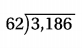The given division expression is: 3,186 ÷ 62
Now,
By using the Long Division,Hence, from the above,
We can conclude that
The value of the given division expression is: 51 R 24

Question 3.
885 ÷ 57
The given division expression is: 885 ÷ 57
Now,
By using the Long Division,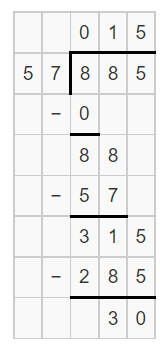Hence, from the above,
We can conclude that
The value of the given division expression is: 15 R 30

Problem Solving

Practice: Copy and Solve Divide. Use partial quotients.

Question 4.
653 ÷ 42
The given division expression is: 653 ÷ 42
Now,
By using the Long Division,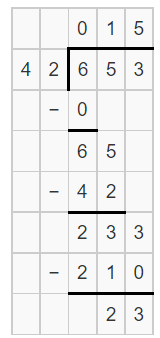Hence, from the above,
We can conclude that
The value of the given division expression is: 15 R 23

Question 5.
946 ÷ 78
The given division expression is: 946 ÷ 78
Now,
By using the Long Division,Hence, from the above,
We can conclude that
The value of the given division expression is: 12 R 10

Question 6.
412 ÷ 18
The given division expression is: 412 ÷ 18
Now,
By using the Long Division,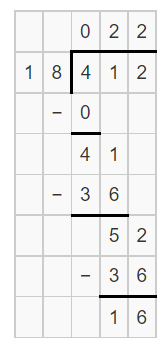Hence, from the above,
We can conclude that
The value of the given division expression is: 22 R 16

Question 7.
871 ÷ 87
The given division expression is: 871 ÷ 87
Now,
By using the Long Division,Hence, from the above,
We can conclude that
The value of the given division expression is: 10 R 1

Question 8.
1,544 ÷ 34
The given division expression is: 1,544 ÷ 34
Now,
By using the Long Division,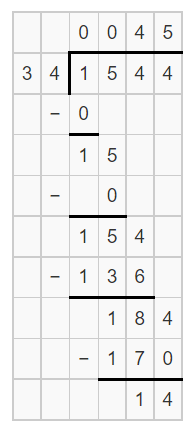Hence, from the above,
We can conclude that
The value of the given division expression is: 45 R 14

Question 9.
2548 ÷ 52
The given division expression is: 2,548 ÷ 52
Now,
By using the Long Division,Hence, from the above,
We can conclude that
The value of the given division expression is: 49

Question 10.
2,740 ÷ 83
The given division expression is: 2,740 ÷ 83
Now,
By using the Long Division,Hence, from the above,
We can conclude that
The value of the given division expression is: 33 R 1

Question 11.
4,135 ÷ 66
The given division expression is: 4,135 ÷ 66
Now,
By using the Long Division,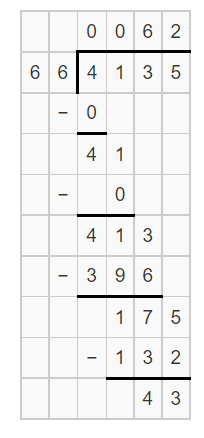Hence, from the above,
We can conclude that
The value of the given division expression is: 62 R 43

Question 12.
H.O.T. What is the greatest possible whole-number remainder if you divide any number by 23? Explain.
The greatest possible whole number remainder would be 22.
Hence, from the above,
We can conclude that
The greatest possible whole number remainder, if you divide any number by 23, would be 22.

Problem Solving

Use the table to solve 13-16.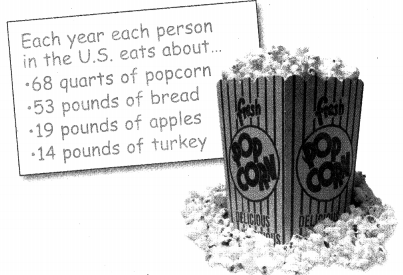Question 13.
How many years would it take for a person in the United States to eat 1,120 pounds of turkey?
The given table is: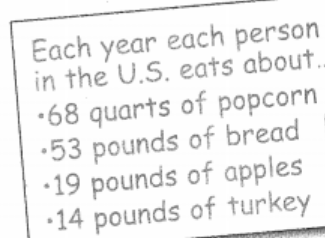Now,
From the given table,
We can observe that
The number of pounds of turkey each person in the U.S eats is: 14 pounds
So,
The number of years would it take for a person to eat 1,120 pounds of turkey = 1,120 ÷ 14
Now,
By using the Long Division,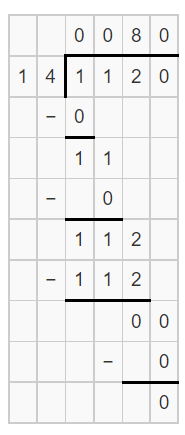Hence, from the above,
We can conclude that
The number of years would it take for a person to eat 1,120 pounds of turkey is: 80 years

Question 14.
Multi-Step If 6 people in the United States each eat the average amount of popcorn for 5 years, how many quarts of popcorn will they eat?
It is given that
6 people in the United States each eat the average amount of popcorn for 5 years
Now,
The given table is:Now,
From the given table,
We can observe that
The number of quarts of popcorn each person in the U.S will eat is: 68 quarts
So,
According to the given information,
The number of quarts of popcorn will 6 persons eat in 5 years = 6 × 5 × 68
= 30 × 68
= 2,040 quarts
Hence, from the above,
We can conclude that
The number of quarts of popcorn will 6 persons eat in 5 years is: 2,040 quarts

Question 15.
H.O.T. Multi-Step In a study, 9 people ate a total of 1,566 pounds of potatoes in 2 years. If each person ate the same amount each year, how many pounds of potatoes did each person eat in 1 year?It is given that
In a study, 9 people ate a total of 1,566 pounds of potatoes in 2 years and each person ate the same amount each year
So,
The number of pounds of potatoes each person eats in 1 year = 1,566 ÷ 9
Now,
By using the Long Division,Hence, from the above,
We can conclude that
The number of pounds of potatoes each person eats in 1 year is: 174 pounds

Question 16.
Write Math Sense or Nonsense? In the United Show Your Work States, a person eats more than 40,000 pounds of bread in a lifetime if he or she lives to be 80 years old. Does this statement make sense, or is it nonsense? Explain.
It is given that
In the United Show Your Work States, a person eats more than 40,000 pounds of bread in a lifetime if he or she lives to be 80 years old
Now,
The given table is:Now,
From the given table,
We can observe that
The number of pounds of bread each person in the U.S will eat is: 53 pounds
So,
The number of pounds of bread each person will eat in 80 years = 80 × 53
= 4,240 pounds
Hence, from the above,
We can conclude that
The given statement is nonsense

Question 17.
You want to design a new calendar system. You decide that there will be 384 days in one year. Each month will have 24 days. How many months will be in one year in your calendar system?
(A) 18
(B) 16
(C) 17
(D) 15
It is given that
You want to design a new calendar system. You decide that there will be 384 days in one year. Each month will have 24 days
So,
According to the given information,
The number of months that will be present in one year in your calendar system = 384 ÷ 24
Now,
By using the Long Division,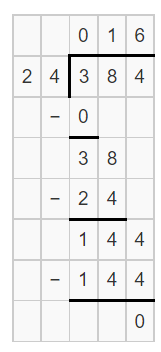Hence, from the above,
We can conclude that
The number of months that will be present in one year in your calendar system is:Question 18.
Analyze David Blaine set a world record for holding his breath for 1,024 seconds. Which partial quotients can you use to find out how many minutes he held his breath (1 minute = 60 seconds)?
(A) 5 + 5 + 2
(B) 10 + 5 + 2
(C) 20 + 5 + 2
(D) 20 + 2
It is given that
David Blaine set a world record for holding his breath for 1,024 seconds
So,
The number of minutes David Blane held his breath = 1,024 ÷ 60
Now,
By using the partial Quotients,
1,024 ÷ 60
= (600 + 300 + 120 + 4) ÷ 60
= (10 + 5 + 2) + (4 ÷ 60)
Hence, from the above,
We can conclude that
The partial quotients that you can use to find out how the number of minutes David Blane held his breath is:Question 19.
Multi-Step The fifth grade is having a picnic this Friday. There will be 182 students and 274 adults. Each table seats 12 people. How many tables are needed?
(A) 23
(B) 15
(C) 11
(D) 38
It is given that
The fifth grade is having a picnic this Friday. There will be 182 students and 274 adults. Each table seats 12 people
So,
The number of tables that are needed = (182 + 274) ÷ 12
= 456 ÷ 12
Now,
By using the Long Division,Hence, from the above,
We can conclude that
The number of tables that are needed is: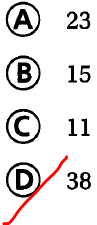Texas Test Prep

Question 20.
The school auditorium has 448 seats arranged in 32 equal rows. How many seats are in each row?
(A) 480
(B) 14,336
(C) 416
(D) 14
It is given that
The school auditorium has 448 seats arranged in 32 equal rows
Now,
The number of seats that are present in each row = 448 ÷ 32
Now,
By using the Long Division,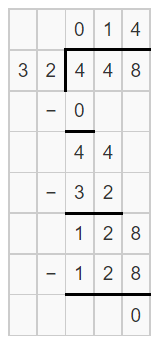Hence, from the above,
We can conclude that
The number of seats that are present in each row is:### Texas Go Math Grade 5 Lesson 2.4 Homework and Practice Answer Key

Divide. Use partial quotients.

Question 1.
723 ÷ 34
The given division expression is: 723 ÷ 34
Now,
By using the Long Division,Hence, from the above,
We can conclude that
The value of the given expression is: 21 R 9

Question 2.
372 ÷ 22
The given division expression is: 372 ÷ 22
Now,
By using the Long division,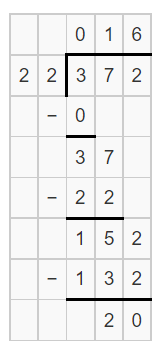Hence, from the above,
We can conclude that
The value of the given expression is: 18 R 20

Question 3.
682 ÷ 31
The given division expression is: 682 ÷ 31
Now,
By using the Long division,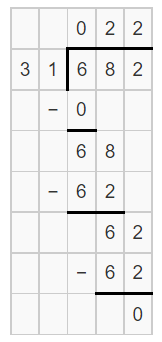Hence, from the above,
We can conclude that
The value of the given expression is: 22

Question 4.
290 ÷ 52
The given division expression is: 290 ÷ 52
Now,
By using the Long division,Hence, from the above,
We can conclude that
The value of the given expression is: 5 R 30

Question 5.
284 ÷ 32
The given division expression is: 284 ÷ 32
Now,
By using the Long division,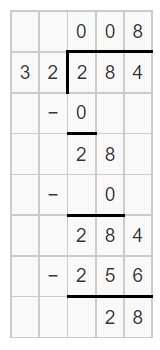Hence, from the above,
We can conclude that
The value of the given expression is: 8 R 28

Question 6.
672 ÷ 15
The given division expression is: 672 ÷ 15
Now,
By using the Long division,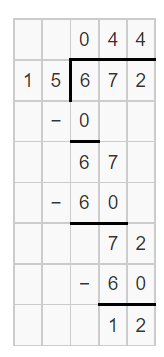Hence, from the above,
We can conclude that
The value of the given expression is: 44 R 12

Question 7.
643 ÷ 35
The given division expression is: 643 ÷ 35
Now,
By using the Long Division,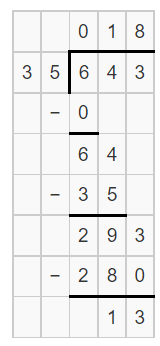Hence, from the above,
We can conclude that
The value of the given expression is: 18 R 13

Question 8.
816 ÷ 62
The given division expression is: 816 ÷ 62
Now,
By using the Long Division,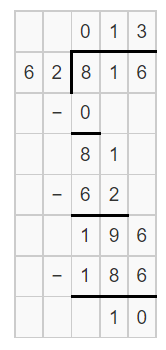Hence, from the above,
We can conclude that
The value of the given expression is: 13 R 10

Question 9.
781 ÷ 26
The given division expression is: 781 ÷ 26
Now,
By using the Long Division,Hence, from the above,
We can conclude that
The value of the given expression is: 30 R 1

Question 10.
283 ÷ 66
The given division expression is: 283 ÷ 66
Now,
By using the Long Division,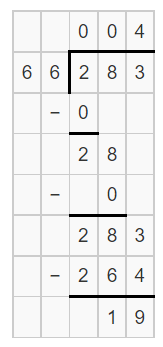Hence, from the above,
We can conclude that
The value of the given expression is: 4 R 19

Question 11.
754 ÷ 23
The given division expression is: 754 ÷ 23
Now,
By using the Long Division,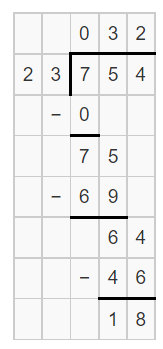Hence, from the above,
We can conclude that
The value of the given expression is: 32 R 18

Question 12.
855 ÷ 36
The given division expression is: 855 ÷ 36
Now,
By using the Long division,Hence, from the above,
We can conclude that
The value of the given expression is: 23 R 27

Question 13.
What is the greatest possible whole-number remainder if you divide any number by 41? Explain.
The greatest possible whole number remainder would be 40.
Hence, from the above,
We can conclude that
The greatest possible whole number remainder, if you divide any number by 41, would be 40.

Problem Solving

Question 14.
The PTA is ordering planners for each of the 154 fifth-grade students. The planners come in packages of 6. How many packages will the PTA need to order? Explain.
It is given that
The PTA is ordering planners for each of the 154 fifth-grade students. The planners come in packages of 6
So,
The number of packages the PTA will need to order = 154 ÷ 6
Now,
By using the Long Division,Hence, from the above,
We can conclude that
The number of packages the PTA will need to order is about 26 packages

Question 15.
Mr. Lee’s science class needs 250 large clips for a project. The clips come in boxes of 24. How many boxes of clips will the class need for the project? Explain.
It is given that
Mr. Lee’s science class needs 250 large clips for a project. The clips come in boxes of 24
So,
The number of boxes of clips the class will need for the project = 250 ÷ 24
Now,
By using the Long Division,Hence, from the above,
We can conclude that
The number of boxes of clips the class will need for the project is about 11 boxes of clips

Lesson Check

Question 16.
Sierra uses 468 golf balls in a display at the sporting goods store. She uses 47 golf balls in each display case. Which answer shows the number of display cases she fills and the number of balls left over?
(A) 8 r2
(B) 9 r13
(C) 8 r31
(D) 9 r45
It is given that
Sierra uses 468 golf balls in a display at the sporting goods store. She uses 47 golf balls in each display case
So,
The number of display cases filled = 468 ÷ 47
Now,
By using the Long Division,Hence, from the above,
We can conclude that
The number of display cases filled and the number of balls leftover is:Question 17.
Kaj has 1,342 marbles in his collection. How many bags does he need if he Is going to put 42 marbles In each bag, and have one with fewer marbles?
(A) 32
(B) 29
(C) 31
(D) 29
It is given that
Kaj has 1,342 marbles in his collection
Now,
According to the given information,
The number of bags kaj will need to put all marbles = 1,342 ÷ 42
Now,
By using the Long division,Hence, from the above,
We can conclude that
The number of bags kaj will need to put all marbles is about: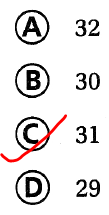Question 18.
Hal has 558 cans of soup to put on a shell. The shelf can hold 72 cans in each row. How many rows can Hal make with the soup cans?
(A) exactly 7 rows
(B) fewer than 7 rows
(C) more than 7 rows
(D) exactly 6 rows
It is given that
Hal has 558 cans of soup to put on a shell. The shelf can hold 72 cans in each row
So,
The number of rows can Hal make with the soup cans = 558 ÷ 72
Now,
By using the Long Division,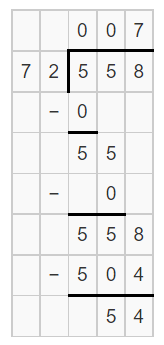Hence, from the above,
We can conclude that
The number of rows can Hal make with the soup cans is: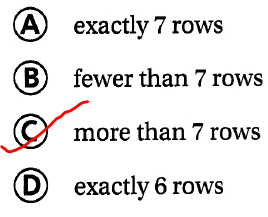Question 19.
Mr. Amos has a bill of $1,260 to pay. He will make 21 equal payments. How much will each payment be? (A)$61
(B) $60 (C)$6
(D) $600 Answer: It is given that Mr. Amos has a bill of$1,260 to pay. He will make 21 equal payments
So,
The amount Mr. Amos will pay for each amount = \$2,160 ÷ 21
Now,
By using the Long Division,Hence, from the above,
We can conclude that
The amount Mr. Amos will pay for each amount is: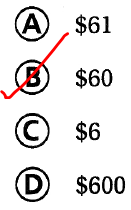Question 20.
Multi-Step Max delivers 8,520 pieces of mail in one year. How many pieces of mail are delivered In two months?
(A) 710
(B) 1,420
(C) 7,200
(D) 700
It is given that
Max delivers 8,520 pieces of mail in one year
So,
The number of pieces of mail delivered each month = 8,520 ÷ 12
Now,
By using the Long Division,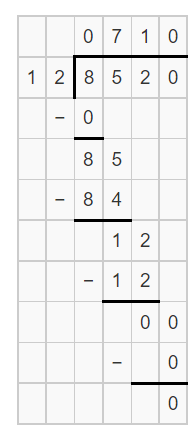So,
The number of pieces of mail delivered in 2 months = 710 × 2
= 1,420
Hence, from the above,
We can conclude that
The number of pieces of mail delivered in 2 months is: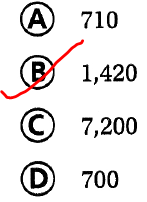Question 21.
Multi-Step The principal is arranging school buses for the spring field trip. There will be 109 students and 17 adults going on the trip. Each bus holds 54 people. How many buses will the group need?
(A) 4
(B) 5
(C) 3
(D) 2
It is given that
The principal is arranging school buses for the spring field trip. There will be 109 students and 17 adults going on the trip. Each bus holds 54 people
So,
The number of buses will the group need = (109 + 17) ÷ 54
= 126 ÷ 54
Now,
By using the Long Division,Hence, from the above,
We can conclude that
The number of buses will the group need is:Scroll to Top